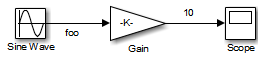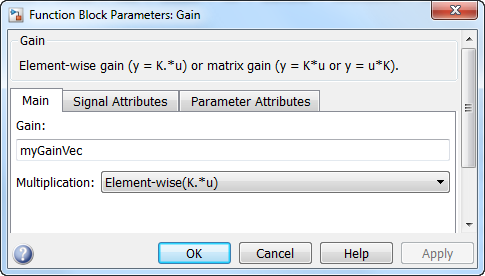## Configure Loop Unrolling Threshold

The Loop unrolling threshold parameter on the Optimization pane determines when a wide signal or parameter should be wrapped into a `for` loop and when it should be generated as a separate statement for each element of the signal. The default threshold value is 5.

For example, consider the model below:The gain parameter of the Gain block is the vector `myGainVec`.Assume that the loop unrolling threshold value is set to the default, 5.

If `myGainVec` is declared as

`myGainVec = [1:10];`

an array of 10 elements, `myGainVec_P.Gain_Gain[]`, is declared within the `Parameters_model` data structure. The size of the gain array exceeds the loop unrolling threshold. Therefore, the code generated for the Gain block iterates over the array in a `for` loop, as shown in the following code:

```{ int32_T i1; /* Gain: '<Root>/Gain' */ for(i1=0; i1<10; i1++) { myGainVec_B.Gain_f[i1] = rtb_foo *         myGainVec_P.Gain_Gain[i1]; } }```

If `myGainVec` is declared as

`myGainVec = [1:3];`

an array of three elements, `myGainVec_P.Gain_Gain[]`, is declared within the `Parameters` data structure. The size of the gain array is below the loop unrolling threshold. The generated code consists of inline references to each element of the array, as in the code below.

```/* Gain: '<Root>/Gain' */ myGainVec_B.Gain_f = rtb_foo * myGainVec_P.Gain_Gain; myGainVec_B.Gain_f = rtb_foo * myGainVec_P.Gain_Gain; myGainVec_B.Gain_f = rtb_foo * myGainVec_P.Gain_Gain;```

See Explore Variable Names and Loop Rolling for more information on loop rolling.

Note

When a model includes Stateflow® charts or MATLAB Function blocks, you can apply a set of Stateflow optimizations on the Optimization pane. The settings you select for the Stateflow options also apply to MATLAB Function blocks in the model. This is because the MATLAB Function blocks and Stateflow charts are built on top of the same technology and share a code base. You do not need a Stateflow license to use MATLAB Function blocks.

Note

If your MATLAB Function block contains code with two nested `for`-loops:

• If the number of iterations of the inner loop is less than the threshold, the code generator first unrolls the inner loop. Subsequently, if the product of the number of iterations of the two loops is also less than the threshold, the code generator unrolls the outer loop. Otherwise the code generator produces the outer `for`-loop.

• If the number of iterations of the inner loop is equal to or greater than the threshold, the code generator produces both `for`-loops

• For an N-Dimensional array the code generator unrolls each contiguous loop until the product of loop iterations is less than the threshold value.